Skip to content
Related Articles
Class 9 RD Sharma Solutions – Chapter 17 Constructions- Exercise 17.1
• Last Updated : 08 May, 2021

### Question 1. Draw a line segment of length 8.6cm. Bisect it and measure the length of each part.

Solution:

Steps of construction:

Step 1: Draw a line segment AB of 8.6cm.

Step 2: With center A and radius more than 1/2 AB, drawn arcs, one on each side of AB.

Step 3: With centre B and same radius, draw arcs cutting the previous arcs at P and Q.

Step 4: Now join in PQ

So, DC = BC = 4.3cm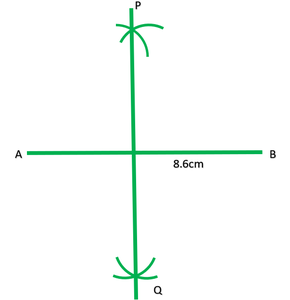### Question 2. Draw a line segment AB of length 5.8cm. Draw the perpendicular bisector of this line segment.

Solution:

Steps of construction:

Step 1: Draw a line segment AB of 5.8cm.

Step 2: With centre A radius more than 1/2 AB draw arcs with one on each side of AB.

Step 3: With centre B and same radius draw arcs cutting the previous arcs at P and Q.

Step 4: Now join PQ.

So, PQ is the perpendicular bisector of AB.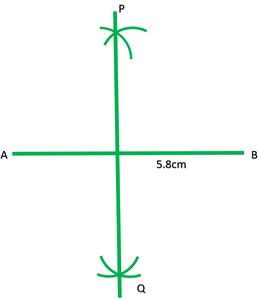### Question 3. Draw a circle with center at point O and radius 5cm. Draw its chord AB, the perpendicular bisector of line segment AB. Does it pass through the center of the circle?

Solution:

Steps of construction:

Step 1: With centre O and radius 5cm draw a circle.

Step 2: Now draw a chord AB.

Step 3: With centre A and radius more than 1/2 AB draw arcs one on each side O.

Step 4: With centre B and same radius draw arcs cutting previous arcs at P and Q.

Step 5: Now join point P and Q.

So, the perpendicular bisector PQ of chord AB passes through the center of the circle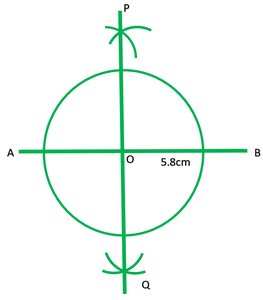### Question 4. Draw a circle with center at point O. Draw its two chords AB and CD such that AB is not parallel to CD. Draw the perpendicular bisector of AB and CD. At what point do they intersect?

Solution:

Steps of construction:

Step 1: With centre O and any radiu, draw a circle.

Step 2: Draw two chords AB and CD.

Step 3: With centre A and radius more than 1/2 AB draw arcs, one on each side of AB.

Step 4: With centre B and same radius draw arcs cutting previous arcs at P and Q respective.

Step 5: Join PQ.

Step 6: With centre B and radius more than 1/2 DC draw arcs one on each side of DC.

Step 7: With centre C and same radius, draw arcs cutting previous arcs at R and S.

Step 8: Now join RS.

So, Both perpendicular bisectors PQ and RS intersect each other at the centre O of the circle.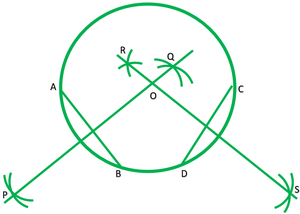### Question 5. Draw a line segment of length 10cm and bisect it. Further, bisect one of the equal parts and measure its length.

Solution:

Steps of construction:

Step 1: Draw a line segment AB of 10cm.

Step 2: With centre A and radius more than 1/2 AB, draw arcs one on each side of AB.

Step 3: With centre B and same radius draw arcs cutting previous arcs at P and Q respectively.

Step 4: Join PQ and which intersect AB at C.

Step 5: With centre A and radius more than 1/2 AC, draw arcs on each side of AC.

Step 6: With centre C and same radius, radius, draw arcs cutting previous arcs at R and S respectively.

Step 7: Now join RS and which intersect AC at D.

So, AD = 2.5cm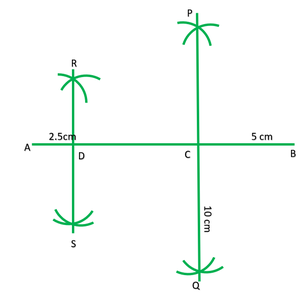### Question 6. Draw a line segment AB bisect it. Bisect one of the equal parts to obtain a line segment of length 1/4(AB).

Solution:

Steps of construction:

Step 1: Draw a line segment AB.

Step 2:. With cetre A and radius more than 1/2 AB, draw arcs one on each side of AB.

Step 3: With centre B and same radius, draw arcs cutting previous arcs at P and Q.

Step 4: Join PQ which intersects AB at C.

Step 5: With centre A and radius more than 1/2 AC, draw arcs one on each side of AC.

Step 6: Which centre C and same radius, draw arcs cutting previous arcs at R and S.

Step 7: Now join RS Which intersect AC at D.

So, AD = 1/4AB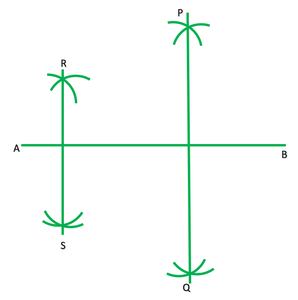### Question 7. Draw a line segment AB and by ruler and compasses, obtain a line segment of length 3/4(AB).

Solution:

Steps of construction:

Step 1: Draw a line segment AB.

Step 2: With centre A and radius more than 1/2 AB, draw arcs one on each side of AB.

Step 3: With centre B and same radius draw arcs cutting previous arcs at P and Q.

Step 4: Join PQ which intersect AB at C.

Step 5: With centre C and radius more than 1/2 CB, draw arcs, one on each side of CB.

Step 6: With centre B and same radius draw arcs cutting previous arcs at R and S.

Step 7: Join RS which intersect CB at D.

So, AD = 3/4 AB.My Personal Notes arrow_drop_up